# Common Data Types used in SQL Server

In this video we’ll walk you though the common data types used in SQL Server; you’ll see examples of each type’s values, and how to define them.

Once you have watched the video check out the sample code below.  I’ve also included a transcript for you to use.

## This is an SQL Minute on What are Common Data Types used in SQL Server?

Transcript:

Welcome to another Essential SQL Minute. In today’s episode, we’re going to talk about the common data types used in SQL server. So the first data type that’s used, and there’s going to be seven that we go over here, is the integer data type.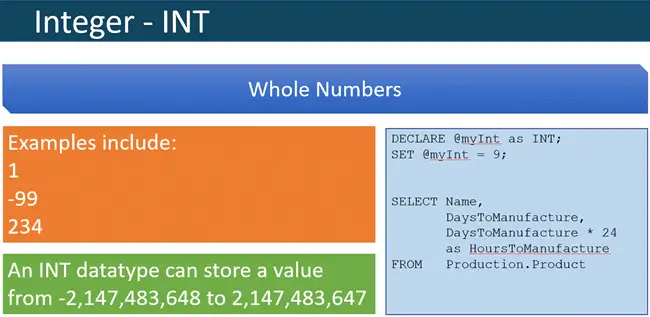The integer data type is declared as a keyword INT. Now, integers are whole numbers. Examples include values such as 1 or -99, 234. They’re essentially numbers that don’t have decimal points. An integer can store a very small number such as negative two billion all the way up to two billion. You can see where we have an example where we declare an integer.

So I’m declaring @myInt as an integer. Then I’m setting myInt to the value 9.

```DECLARE @myDate as DATETIME;
SET @myDate = '2015-09-15’```

I also have an example of a query where I am taking DaysToManufacture, which is declared as a column of integer data type, and I’m multiplying it by an integer value of 24 to find out how many hours it’s taking to manufacture.

```SELECT Name,
DaysToManufacture,
DaysToManufacture * 24
as HoursToManufacture
FROM   Production.Product```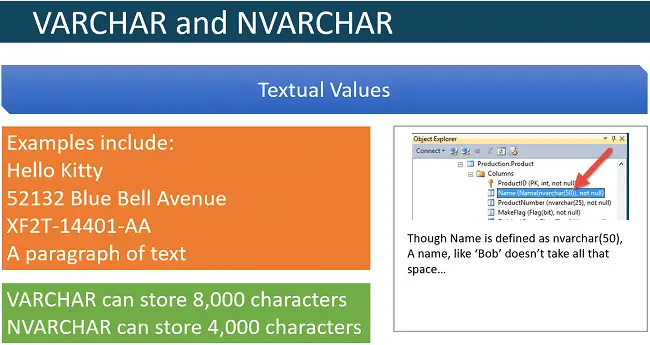The next two common data types I want to go over are VARCHAR and NVARCHAR.

So, VARCHAR is used to store textual data, and NVARCHAR is used to store Unicode data.

Unicode data is extended textual data. It’s essentially fancy data, usually foreign characters. It’s two bytes per value.

That’s why VARCHAR stores 8,000 characters, but a NVARCHAR can only store 4,000 characters, because NVARCHAR’s using two bytes per value.

Examples of textual values would be like “Hello Kitty” or an address such as like “52132 Blue Bell Avenue.” It could be like a part number “XF2T-14401-AA;” This is a Ford part number. Or it could be a paragraph of text.  Here you can see where we have defined nvarchar(50) from the AdventureWorks database.

I think it’s important to point out, with VARCHAR and NVARCHAR, that even though a name could be defined as character 50, if you store a short name in the column such as Bob, I won’t take up all 50 characters.

So it’s not going to be like Bob and then 47 spaces. So, the nice thing about VARCHAR is it only takes up as much space approximately as what you put into it.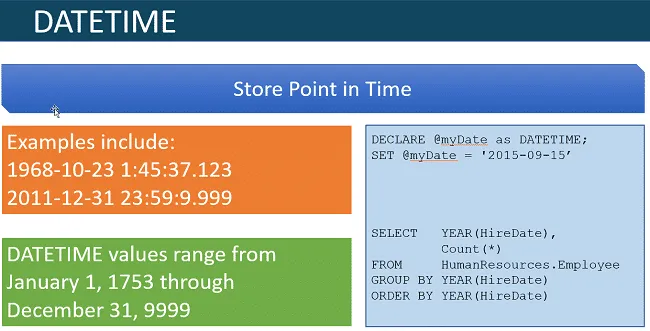The next common data type we’re going to go over is DATETIME. This is used to store a point in time. Examples would include “October 23, 1968 at 1:45” in the morning. You can see here it’s showing the hours, the minutes, the seconds and then hundredths of seconds … or thousandths of seconds, that is.

Here’s another example of the last possible moment before 2012. So this is December 31st almost at midnight. So DATETIME’s can range from January 1, 1753 through December 31 19 … or I guess … 9999.

So, for contemporary dates, I think DATETIME fits perfectly. However, if you’re writing an application for history and you need to work, for instance, dates from the Egyptian times, you may need to come up with a different way of storing that information, because, as you see, our DATETIME only goes back to the year 1753.

So here’s an example of declaring a DATETIME. You see we’re setting the date to DATETIME, and we can use single quotes and put our date, and in single quotes as year dash month and then day, and it will implicitly get converted to DATETIME.

```DECLARE @myDate as DATETIME;
SET @myDate = '2015-09-15’```

This DATETIME, here, curiously enough, is going to have zero, zero, zero, zero, zero, zero as the hours, minutes and seconds. So it’ll be implied on the date (e.g. 2015-09-15 00:00:00)

The nice thing about using a DATETIME data type is, is that then you’re able to use built-in functions that operate on DATETIME such as year. Here would be an example of a query that would go out and summarize by year the number of employees that were hired.

```SELECT   YEAR(HireDate),
Count(*)
FROM     HumanResources.Employee
GROUP BY YEAR(HireDate)
ORDER BY YEAR(HireDate)```A DECIMAL data type is used to show fractional values, so those that include decimals. What sets the DECIMAL data type apart from another data type called FLOAT is that the DECIMAL data type is very good for financial applications.

You’ll see that DECIMAL data types are defined with a precision and scale.

This gives them a very good exact nature. And you can see some examples where I have defined DECIMAL(8,3), meaning I have eight total digits and a scale of three.

There’s some examples of digits here where you’ll see where I have five in the whole numbers position and then three in the decimal for a total of eight.

So the rule of thumb here really is, is that, the precision has to be less than 38 digits and so does the scale. So the scales can be less than the precision, and the precision is going to be less than or equal to 38 digits.

Here’s an example of declaring a decimal. Here at seven total digits, four of which are the decimal portion.

```DECLARE @myDecimal as DECIMAL(7,4);
SET @myDecimal = 365.3235;```

One thing to point out is that if I had set this variable to, let’s say, 3,651.3235, I would get an overflow error, because I’ve only defined it to have three places in the whole number.

If I try to define the variable or assign it with four, it’ll overflow. One thing to point out when using decimals, or floats for that matter, is that when you specify them in your SQL, you need to put the decimal place in your statements.

```SELECT   DISTINCT Weight
FROM     Production.Product
WHERE    Weight
BETWEEN  29.00 and 189.00
ORDER BY Weight DESC```

So, here, you see I just don’t say between 29 and 189; I’m saying between 29.00 and 189.00. That helps signify that as a decimal.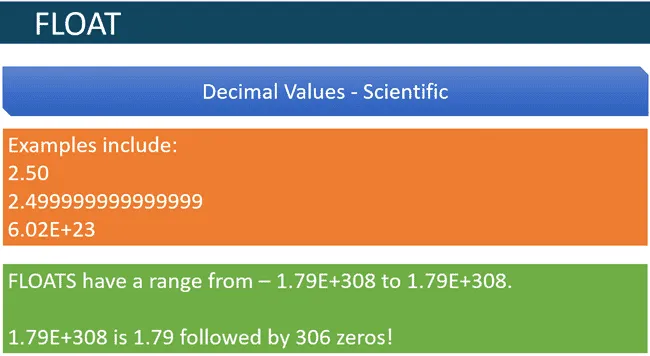So a companion to the DECIMAL data type is the FLOAT data type, and this is a really good data type to use for decimal values when you’re doing with scientific values.

The reason is, is that, the decimal can be used for a large range of numbers. It can’t handle the magnitude of numbers that float can.

If you look here, float can handle all the way up to 306 zeros. So, basically, 1.79 times 10 to the 308th power. So it’d be like 179 followed by 306 zeros.

Here it is…

179000000000000000000000000000000000000000000000000000000000000000000000000000000000000000000000000000000000000000000000000000000000000000000000000000000000000000000000000000000000000000000000000000000000000000000000000000000000000000000000000000000000000000000000000000000000000000000000000000000000000000000

So, it’s a very, very large number. For example, here’s Avogadro’s number (6.02 E23), which is the number of atoms in a mole. It’s way past the trillions. A very large number.

Other examples of FLOAT are 2.50, or what I wanted to point out is in some cases when you enter 2.50, internally, the number won’t get represented exactly as 2.50 but maybe as 2.499999.

So, it becomes kind of tricky when you’re comparing values with FLOAT, as the internal representation may be that one decimal place off.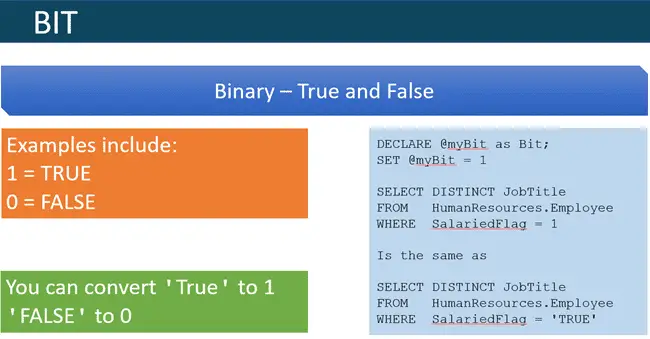The last data type I want to cover is the BIT data type. This is used to signify yes or no, or true or false. Examples include 1 for true and 0 for false.

You can convert a true text value into a 1, and false will convert to 0.

```DECLARE @myBit as Bit;
SET @myBit = 1```

So, here I have an example where I am getting all job titles from human resources where the SalaryFlagged is 1.

```SELECT DISTINCT JobTitle
FROM   HumanResources.Employee
WHERE  SalariedFlag = 1```

This is the same thing as me selecting from the same query where the SalaryFlagged is true, and it’ll implicitly take this true here and convert it to a 1, because it knows that this is a BIT, and so, it’s going to convert this data type to 1.

```SELECT DISTINCT JobTitle
FROM   HumanResources.Employee
WHERE  SalariedFlag = 'TRUE'```

I declare a variable as BIT, and then I could just set that bit to 1 or 0. So these are the main data types that are used in SQL.

#### Whats Next

There are other variations, such as esoteric data types. I would encourage you to go study them, but by and large, if you learn these seven common data types, these are the ones that you need to know for the 70-761 exam and essentially are the main data types that you could use today to design and construct your database.

#### Kris Wenzel

Kris Wenzel has been working with databases over the past 30 years as a developer, analyst, and DBA. He has a BSE in Computer Engineering from the University of Michigan and a MBA from the University of Notre Dame. Kris has written hundreds of blog articles and many online courses. He loves helping others learn SQL.